## 2009-02-26

### lambda and name

Japanese version

In mathematics, we use function names as f, g, and so force. When we have more than one function, these names f and g are useful to distinguish them. I assume the name f comes from English ``function'' in English (an old language on a plant called Earth.) But for any function we made, we wrote it as f:= ... except very special functions. We always wrote f:= ... for functions, for example, f(x):= x, f(x):= x^{2}, f(x):= sin(x), and so on.

It does not matter to write f:= ... or g:= ... . The substance part is this ... part, f is just like a tag of a parcel. If we can get a parcel, the tag does not matter.

Every planet has city halls if there are governments. Every planet has banks if there exists money. The people living these planets must wait in a long queue to get the service. This is usually defined by a law. If these services violate the law, i.e., they did not make the people wait, they will be arrested. Surely your planet would be the same. Most of the city hall in these planets issues a number on a card as describing a waiting order. A function name is just like such a number on the card. There is not so much meaning.

I prefer to be called by my name when I am waiting for my turn. But I will be fine, if this call-by-number system makes less waiting time than the call-by-personal-name system. The important thing is this ``number'' indicates what or who. If ``10'' indicates me, I am ``10.'' I have no feeling to prefer number 10. I do not say ``Oh, the number 10? I do not accept that number.'' The important thing is ``mapping'' from the number to me. Therefore, I could accept any number like 10, 42, or 156.

Although I repeated a name is a second matter, mapping from name to substance is essential. I could say a name is for mapping. If you can say several things by one name, it is a great first step of abstraction.

But, lambda calculus avoids names. If we do have less names, we can concentrate the substance. We use the name ``lambda'' instead of ``function''. ``Lambda'' seems no meaning, dry, and it seems not a name. On the other hand, a name is important for human understanding process.

Marvin: ``What a contradiction! A name is important, but does not matter. I'm depressed.''

I mean, mapping is the substance, which is depends on name. Therefore, a name is important ``for human,'' but the name itself can be anything like 10. In this sense, it does not matter. Isn't it clear?

A computer called a computer in English, Keisanki in Japanese, Rechner in German. Even the real substance computer hardware is the same, it is called different, different names. 1 is one in English, Eins in German, and ichi in Japanese. ``A rose by any other name would smell as sweet.'' ``one by any other name should share the same concept as 1.'' Human usually understand a substance by its name. In this sense, the name is important. Some can think this is a philosophical problem. But a computer language called scheme (a lisp) clearly distinguish the substance and its name. The substance is lambda, and we can call it directory, or we can bind the name to a lambda (mapping!), then call it by the bind name. This is well defined. A machine can perform it. So, I think it is not a problem like ``what is life?'' Of course, it is difficult to make a map with a good name. Maybe think about a good name is a philosophical problem. (Here I use a stereotype as philosophy == difficult).

## 2009-02-22

### function = lambda

Japanese version

The first my problem about lambda calculus is that there is only one function, lambda. Actually, lambda means function, therefore, it is natural to call function as ``function'' -- lambda. This sounded strange for me. But lambda calculus's function is a bit different from the conventional function at that time, I assume they needed a name to distinguish them.

Anyway, function is lambda. I don't know that why the symbol ``lambda'' is selected as representing function. There are several hypotheses, but we don't exploit it here. (One hypothesis is the lambda calculus is first started in logic. Greek `L' is lambda. But actually I don't know, so it is nice way to write that ``We don't exploit it here.'')

My second trouble was why ``calculus?'' lambda calculus seems more like Algebra. In symbolic logic, these are called calculus. However, I am a amateur mathematician, I do not know about this also.

### Abstraction --- Infinite can live in finite (2)

Japanese version

Human has an ability to abstract each concrete numbers, like, 1, 2, 3, ... to ``(general) numbers'' by name. And we can understand that they (numbers) can include all possible numbers. This is outstanding ability of human. It is enough interesting for me that any people have this counting ability and ability of speak except in case of diseases. But a machine seems not have such ability (yet).

Human can easily perform this abstraction. For example, human can have a concept of ``chairs.'' It is still a difficult problem to search pictures of chairs. Human can see a catalog book of IKEA (a famous furniture shop now in 2009) and can recognize chairs. How can we use it is also easy to be understood. A word ``chair'' includes infinite type of chairs. But, a human can recognize a new design chair of this year which never existed until this year.

Similarly, lambda calculus does not treat each concrete function. It concerns all kind of functions. We can never predicted what kind of problem we need solve in the future. However, if we have a theory for any kind of functions and if this concept ``function'' is enough abstracted, we can use this theory to solve the problem arisen in the future. Mathematics is hard to be obsolete. For example, I doubt the idea of ``addition'' would be obsolete later in thousand years. The reason we think about this lambda calculus or abstraction is we expect the theory last long. I like a knowledge which last long since I do not need to learn all the time. Many study/science aimed this way, but it is not so easy.

Mathematics sticks at infinity, definition, or something mathematics stuffs since we want to expect that what correct today is also correct tomorrow. It is interesting for me that mathematics is the basics of almost all sciences and technology. A study, which does not change once it is established, drives all the world technology change. But if you think a bit more, this is natural. The advancement of technology is based on yesterday's advancement. If this base is changed every day, we could have never advance. The technology itself can change every day. But the base of technology can not be unstable. The basis of the technology is so stable, therefore, we can put some change based on that.

## 2009-02-19

### Abstraction --- Infinite can live in finite (1)

Japanese version

Here I would like to remind you about abstraction. Abstraction derives a common idea that is not associated with any specific instance from many ideas associated with instances. Because this blog is oriented to ``getting a feeling of understanding,'' I need to explain the motivation, ``why we should need to mention about abstraction?'' We introduced the idea, abstraction, because concrete instances are not sufficient. If someone can understand about what is the addition, s/he can add a pair of numbers out from infinite combination of numbers. Because any memory devices have a limit in size, there is a limit to add the numbers in finite combination. It is a strong idea that understanding we can add any number.

We could design a machine that seems compute numbers by memorize the answers. This is a different approach to make machine to compute. We could teach 1 + 1 equals 2, 1 + 2 equals 3. Then if you ask this machine, what is 1 + 2? Then you can ask to the machine, what is 2 + 3. The machine can not answer the question since the machine has not memorized that answer for a long time. This is why remembering each instance is not sufficient.

We could abstract 1, 2, 3, ... as a concept ``numbers.'' We could design a machine which process ``numbers.'' This means a machine can treat infinite kind of number instances.

Here infinity means, we can add ``any number'' which is quite normal. 1+10 is possible, but it should not happens that 1 + 128 is not possible. Sometimes, I encounter a person who think that ``nothing can do on infinity.'' However, you can chose one number from infinite numbers and you can add it with one. It might be possible your choice is too large and you can not say that number in your life time. However, you know you can add any two numbers.

I personally think that the most important subject in the high school mathematics is differentiation and integration. It has an idea, ``Infinite can live in finite.'' You can see this through the concept called convergence. For example, there are infinite number of numbers between zero to one. We can project and create one to one mapping from an infinite plane to hemi-sphere with radius one except one point. Pythagoras thought infinite can not live in finite, Zenon made a famous paradox to indicate Pythagoras's mistake.

We could think about infinite numbers by an abstraction of number itself. This is a strong idea. In lambda calculus, we abstract functions with three definitions. All three definitions are about functions. Thanks to abstraction, we can handle any functions in lambda calculus --- infinite type of functions.

## 2009-02-16

### The idea of next level

Japanese version

So far, we saw machines that can compute numbers like SUCC1 or Pop1. Each machine executes own procedures. A procedure is a sequence of instructions, for example, what does the machine do on an input, what does the machine do to create an output. Here we intentionally limited that the machine can only execute one instruction at a time for simplicity, means there is no parallel processing. The examples of instruction are: copy the input number on the input table to the output table, remove head and tail from the number on the output table.

We show the possibility of calculation by a machine which executes a procedure. The concrete examples are SUCC1 and Pop1. We can construct arbitrary machines in the same way. But, do we need to design machines for each problem? To compute addition, do we need Pop1? To compute subtraction, do we need Subtraction1? Or, can we design a little bit more general machine, that one machine can do addition, subtraction, multiplication, and division?

When a mathematician reached this point, s/he can not help thinking: ``How many instruction is sufficient to solve all the mathematical problem?'' ``How can we consider 'all' mathematical problem?'' ``What can we compute and can not compute in this way?''

We have already talk about a motivation of the lambda calculus. The motivation of lambda calculus is to think about the foundations of mathematics. Lambda calculus re-think the mathematics by a formal way. This lead us that calculation can be composed of procedures and that are composed of instructions. Each instruction is performed by a machine. Therefore, calculation can be done by a formal way. It is possible! We saw the possibility. Then people start to ask the next level questions. Many of the science or mathematics develed in the similar patterns.

It's time to go to the next level of lambda calculus.

## 2009-02-12

### A machine which executes a procedure ... Pop1 (2)

Japanese version

I show you the instructions of Pop1 at the last session.

Pop1's program is fixed, this means we can not change the program. The Cybernetics coorp. considers their software is top secret matter. Even the people working for the company can not see it. Actually developers of the software can not read that. But, if you run the program, Pop1 displays what instruction is currently executed. So we can just write them down (Figure (I)). (The references (a), (b), (c) are in the last blog.) You may find that Pop1 has only three instructions.

Marvin``It's a waste to have three instructions in such a machine.''

Of course, we could construct a computer with one single instruction, which is Turing equivalent to the any computers we have. But, human needs understandings, Marvin. Please do not forget that. (There are several variations of one instruction set machine. My favorite one instruction computer is in the book, Computer Architecture: a Quantitative Approach.)

1 (a) Copy
2 (b) Delete head and tail
3 (a) Copy
Figure (I) Pop1 program.

If you never wrote a computer program, you might wonder this is a real program or not. This is a real program. All the programs stuffs are composed of small program units (instructions), even the program of the weddings, TV program, Olympic game program, ... are the same way. Pop1 program is like this.

Figure (II) shows the all the procedures. I assume you can see the animation. But in case you can not see the animation or the procedure, I put the all figures also.Figure (II) Pop1 procedure (click on the image to see the animation gif)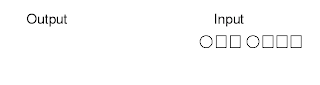Figure (1) Initial stateFigure (2) 1. copy the input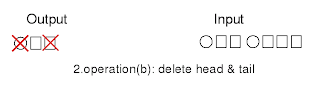Figure (3) 2. delete head and tail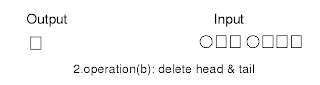Figure (4) 2. delete tail and head resultFigure (5) 3. copy the input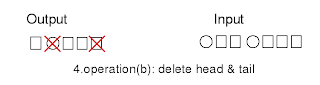Figure (6) 4. delete head and tail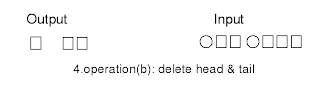Figure (7) 4. delete head and tail resultFigure (8) 5. add head and tailFigure (9) 5. add head and tail result

Now, what does this program? It does not matter the machine does something without meaning ``for human being,'' but I can show something meaning example here. Pop1 gets two inputs A and B, and then outputs one result C. Here A, B, and C are called variables. Maybe someone wonders what is variables? Let's talk about what is variable later if I remember it. I will write this as

Pop1(A, B) -> C.

Set A is 1, B is 2, then the result is

Pop1(1, 2) -> 3.

Pop1 gets input 1 and 2, then the result is 3. Let's see more.

Pop1(0, 0) -> 0
Pop1(0, 1) -> 1
Pop1(0, 2) -> 2

Pop1(1, 0) -> 1
Pop1(1, 1) -> 2
Pop1(1, 2) -> 3

Pop1(2, 0) -> 2
Pop1(2, 1) -> 3
Pop1(2, 2) -> 4

Can you see what is Pop1? This is ``Plus operation.'' Now you see why the machine's name is Pop1 (Plus OPeration 1). The name is for human. It actually does not matter if it is Lambda1, Machine1, Hokuspokus1, or whatever. (I followed the book from Sato and Sakurai.)

Let's rewrite this as in the usual notations.

Pop1(0, 0) -> 0 => 0 + 0 = 0
Pop1(0, 1) -> 1 => 0 + 1 = 1
Pop1(0, 2) -> 2 => 0 + 2 = 2

Pop1(1, 0) -> 1 => 1 + 0 = 1
Pop1(1, 1) -> 2 => 1 + 1 = 2
Pop1(1, 2) -> 3 => 1 + 2 = 3

Pop1(2, 0) -> 2 => 2 + 0 = 2
Pop1(2, 1) -> 3 => 2 + 1 = 3
Pop1(2, 2) -> 4 => 2 + 2 = 4

Finally, we have a computer, a machine that can compute in these days. At the old time, a computer is a person's job. I heard a lot of computer were women. Although this computer has ability to get angry if you mistake the inputs, it does not understand what the number is. It just follows a procedure. However, it ``looks like'' it can compute. This ``Looks like'' is important. I believe nowadays computer (2009) still does not understand what the number is. Computation is a machine procedure. It looks like computing, and the result is correct.

Appearance and contents, name and meaning are different things. But ``formalization'' is an idea if all the appearances are the same, let us think the contents are also the same. This is not a shallow idea. If there are perfect copies, you can not distinguish. Then they are the same. For example, you can buy a software, but each software is a copy. We expect all the software have the same functionality. This is important from the industrial point of view. Of course, exact I am not ready to accept the exact copy of myself -- a copy of human being. But, if I buy two same model computers, I want to have the same. If I buy the same DVD title, I want to have the same. Also I expect the quality should not matter if I bought it from a shop in Berlin or in Frankfurt.

On the other hand, I prefer a hand written mail from my friends since each of them is different. I look for the eternal truth in mathematics, but I love also a limited life. It looks like contradiction. How is the opinion of Marvin, who experienced the universe five times.